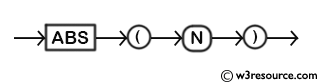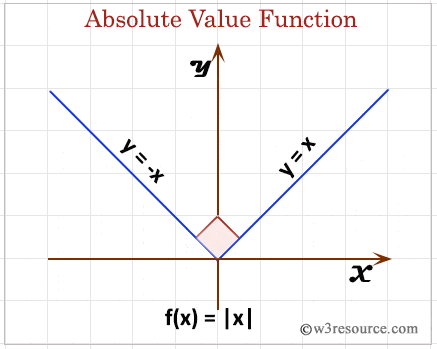# MySQL ABS() function

## ABS() function

MySQL ABS() returns the absolute value of a number.

Syntax:

` ABS(N); `

Argument:

Name Description
N A number whose absolute value is to be retrieved.

Syntax Diagram:MySQL Version: 5.6

Pictorial presentation of MySQL ABS() functionExample:

The following MySQL statement will return the absolute value of a positive number specified in the argument.

Code:

``````SELECT ABS(5);
```
```

Sample Output:

```mysql> SELECT ABS(5);
+--------+
| ABS(5) |
+--------+
|      5 |
+--------+
1 row in set (0.01 sec)
```

Example : ABS() function using negative value

The following MySQL statement will return the absolute value of a negative number defined in the argument.

Code:

``````SELECT ABS(-5);
```
```

Sample Output:

```mysql> SELECT ABS(-5);
+---------+
| ABS(-5) |
+---------+
|       5 |
+---------+
1 row in set (0.01 sec)
```

All Mathematical FunctionsPrevious: UPPER
Next: ACOS()

﻿